Search IntMath
Close

450+ Math Lessons written by Math Professors and Teachers

5 Million+ Students Helped Each Year

1200+ Articles Written by Math Educators and Enthusiasts

Simplifying and Teaching Math for Over 23 Years

# Math of ECGs: Fourier Series

By Murray Bourne, 30 Mar 2010

I recently had a medical checkup that included an ECG (electro-cardiograph).

This is what my ECG looked like:

## How an ECG is done(Left) Electrodes used for an ECG. Image source
(Right) Nurse performing an ECG. Image source

The electrodes are connected to various parts of your anatomy (chest, legs, arms, feet) and voltage differences over time are measured to give the ECG readout.

The horizontal axis of the ECG printout represents time and the vertical axis is the amplitude of the voltage.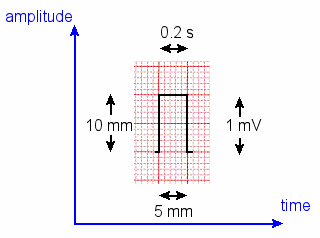Amplitude units are millivolts (mV) and on the graph, 1 mV = 10 mm high.

The time scale is 25 mm = 1 second (or 1 mm per 0.04 seconds on the graph).

So here's my readout for Lead II, representing the voltage between the positive electrode on my left leg and the electrode on my right arm. Each thicker red vertical line represents a time of 1 second.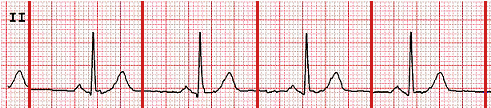Apparently (according to the doctor), this indicates my heart is quite healthy.

In more detail, the features of the repeated pulse we are looking at are as follows.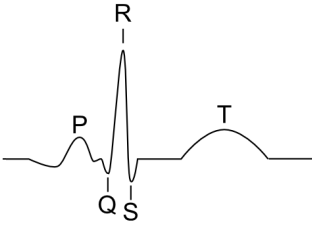[Image source: T. Burke]

The P wave is caused by contraction of the right atrium followed by the left atrium (the chambers at the top of the heart).

The QRS complex represent the point in time when most of the heart muscles are in action, so has highest amplitude.

The T wave represents the polarization of the ventricles (the chambers at the bottom of the heart).Human heart showing atria and ventricles.
[Image by UCSD, source page no longer available]

## Modeling the Heartbeat Using Fourier Series

A heartbeat is roughly regular (if it isn't, it indicates something is wrong). Mathematically, we say something that repeats regularly is periodic.

Such waves can be represented using a Fourier Series.

#### Assumptions

In my case, my heart rate was about 70 beats per minute. For the sake of simplicity, I'll assume 60 beats per minute or 1 per second. So the period = 1 second = 1000 milliseconds.

Also for simplicity, I will only model the R wave for this article. To get a more accurate model for the heartbeat, I would just need to do a similar process for the P, Q, S and T waves and add them to my model.

I observed that my R wave was about 2.5 mV high and lasted for a total of 40 ms. The shape of the R wave is almost triangular and so I could have used straight lines for my model, but these don't give us a smooth curve (especially at the top - it must be continuously differentiable).

A better approach is to use a polynomial (where the ascending and descending lines are close enough to being straight), so my model is as follows (the time units are milliseconds):

f(t) = -0.0000156(t − 20)⁴ + 2.5

f(t) = f(t + 1000)

#### Explanation of the Model

The model is based on a quartic (power 4) since this will give me close to the shape I need (a parabola would be too broad). I'm using similar thinking to what I was doing in this article, where I move a curve around to where I want it.

The (t − 20) term comes from deciding the curve should start at (0,0), (which makes our lives easier), it will pass through (40,0) since the pulse is 40 ms long, and be centered on t = 20.

The "+2.5" comes from the fact the amplitude of the pulse is 2.5 mV.

The -0.0000156 comes from solving the following for a when t = 0.

a(t − 20)⁴ + 2.5 = 0.

The "f(t) = f(t + 1000)" part means the function (pulse in this case) is repeated every 1000 ms.

#### Graph of the Model

This is the graph of part of one period (the part above the t-axis from t = 0 to t = 40):Of course. this is just one pulse. How do we produce a graph that repeats this pulse at regular intervals?

This is where we use Fourier Series.

I'll spare you all the details, but essentially the Fourier Series is an infinite series involving trigonometric terms. When all the terms are added, you get a mathematical model of the original periodic function.

To obtain the Fourier Series, wee need to find the mean value, a0, and 2 coefficient expressions involving n, an and bn which are multiplied by trigonometric terms and summed for n = 1 to infinity.

#### Mean Value Term

a0 is obtained by integration as follows (L is half of the period):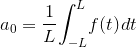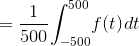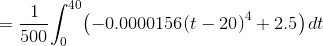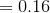(The section of the curve we need for this part of the problem is from t = 0 to t = 40, so that's why we chose those values for the limits of integration in the second last line.)

#### First Coefficient Term, an

Next, we compute an: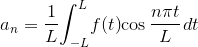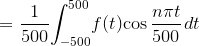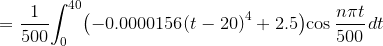The answer for this integral is pretty ugly. I've included it in the PDF solution.

#### Second Coefficient Term, bn

Now for bn: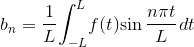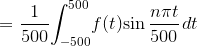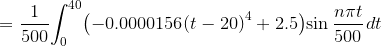Once again, I have spared you from the full details.

Finally, we put it all together and obtain the Fourier Series for our simple model of a heart beat: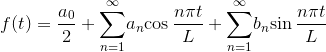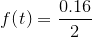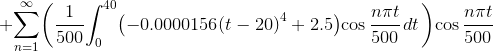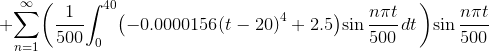When we graph this for just the first 5 terms (n = 1 to 5), we can see the beginnings of a regular 1-second heart beat.The above graph shows the "noise" you get in a Fourier Series expansion, especially if you haven't taken enough terms.

Taking more terms (this time, adding the first 100 terms) gives us the following, and we see we get a reasonable approximation for a regular R wave with period 1 second.I added the T wave for this next model (in blue).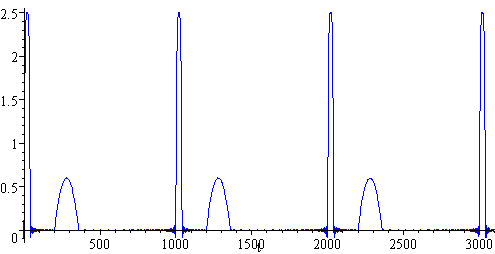I used a parabola for the T wave because the shape of the T wave is broader than the shape of the R wave.

We could keep going, adding the P, Q and S waves to get an even better model.

See the complete solution (up to the T wave, created using Scientific notebook) here:

#### What have we done?

We have taken a single spike representing one R wave of my heartbeat. We then found a formula that repeats our spike at regular time intervals. The Fourier Series (an infinite sum of trigonometric terms) gave us that formula.

Finally, we added the T wave, using the same theory as before.

Fourier Series is very useful in electronics and acoustics, where waveforms are periodic.

For more on Fourier Series go to:

Fourier Series

Don't miss the section on how Fourier is used to create Digital Audio, in

### 54 Comments on “Math of ECGs: Fourier Series”

1. vonjd says:

Just to let u know: "The answer for this integral is pretty ugly. I’ve included it in the PDF solution." - brings up a 404

2. Murray says:

Oops - the second link was fine but I forgot to fix the first. Thanks for your feedback.

3. nahum says:

i liked the easy-to-understand English.It made it not boring.

4. sharma k k says:

it is quite easy to under stand a mathematical approach.

5. gagangc says:

Sir,

i have modelled R curve in different manner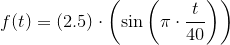for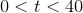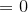for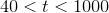and fourier series up to two terms is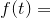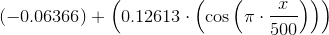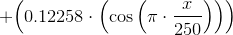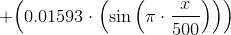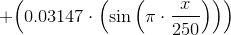when i plotted it i got satisfactory answer as i considered only two terms.

please check my answer and let me know if i am correct.

it took lot of effort and time from me to calculate all coefficients by hand.

6. Murray says:

Hi gagangc. This looks good to me! Good on you for churning away with this.

7. biofredo says:

did you calculate de PRS equations?

8. gagangc says:

Sir,

i have a doubt regarding fourier transform of rectangular function.If FT indicates frequency contents of time domain signal,then FT of rect function is sinc function which have infinite frequencies.Does this mean a simple rect function has infinite frequencies??

9. Murray says:

Hi gagangc. The rectangular function is a single pulse, so it's not relevant to talk about infinite frequencies. These 2 sources may help:
Wikipedia's article
Wolfram Demonstrations (requires a plugin)

Hi Murray,
I'm interested to know how you have generated your last 3 graphs. If possible could you please email me your matlab codes. My email address is [hidden for protection from spam]

thanks

11. Murray says:

@Dan: Two of the last 3 graphs are based on the formulas I developed in the article (I did 5 terms for the first, then added 100 terms for the next.)

The last one adds the T-wave using the same process (I estimated the "blip" above the horizontal axis, then built it into the Fourier series. I suspect the details are hidden in some archive by now.

I'm using Scientific Notebook for these graphs. It allows for graphing of a summation notation expression.

12. Kate says:

Hello! I was curious if you could post your findings for the P, Q, and S waves? I have done it myself, but would love to check if I did it correctly. Many thanks!!

13. Murray says:

@Kate: As I mentioned in the article, I stopped after the T wave.

Basically, in this work, if it looks right, it is (very likely) right! Have you graphed your solution? That usually tells you right away whether you are on the right track. Use any of the online graphers (like Desmos) to graph the first few terms for you.

14. Kate says:

No, I havent graphed it. I was planning on using MatLab to get a graph.
Quick question -- when solving a(t-20)^4+2.5=0 where you got -0.0000156 -- what is a? Or, could you explain this process in more detail? And, why use a quartic for the R wave and a power of 2 when you solved for the T wave? Thank you!!

15. Murray says:

@Kate: I've written some additions to the article which explain your questions. (You may need to refresh the page to see the changes.)

16. hari says:

Sir,

Can u clearly explain me how did u get this function f(t)
as shown below. it wil be very helpful for me if u clarify my doubt.
f(t) = -0.0000156(t − 20)⁴ + 2.5

f(t) = f(t + 1000)

thanq

17. Murray says:

@Hari: Did you go to the article I linked to, How to draw y^2 = x - 2?

I'm using similar thinking to arrive at the function you are asking about.

The minus at front is there to turn the curve "upside down" (so it is n-shaped and not u-shaped).

The "-20" is there because I need to move it over to the right by 20 milliseconds.

It's power 4 because it's more narrow than a parabola (which would be power 2).

The "+2.5" is to move the curve up by 2.5.

I just experimented using this graph facility until I was satisfied.

The f(t) = f(t + 1000) just means "repeat the curve every 1000 milliseconds".

Hope that helps.

18. Successful Android App Development at a Small Scale says:

[…] timings for each phase of the wave (for a mathematical breakdown of the ECG Fourier series, this is a good start). We popped these timings into our code and the result did not disappoint. It’s […]

19. Daniel Manogaran says:

Hi Murray,

Can you please explain why the limits of integration change from [-500, 500] to [0,40]? Also what software did you use to compute those complex integrals, Mathematica doesn't seem to work when I tried, I even entered your expression and got a different value.

Thanks,

Daniel

20. Murray says:

@Daniel: I added an explanation in the post, just under the first time I changed those limits. In the PDF of the complete solution, you'll see I've done a similar thing for the T wave portion, t=200 to t=360.

I was using Scientific Notebook, which at the time used Maple engine. With such examples, the proof is in the pudding - if it looks right, it probably is right! (That is, I wanted a "blip" from 0 to 40 that went up to around 2.5 on the V axis, and that's what I got. You know immediately if your calculations are wrong because the graph will look all wrong.)

Like many trigonometric calculations, the form of the answer could be quite different, but has equivalent value.

All the best with it!

21. hajar says:

what is the partial differential equation for this wave ?
is there?
and The initial value problem marginal?
I want search about partial differential equation and solve it by Fourier Series

22. Phillip says:

Hello

I'm attempting to graph the P wave, and I have a model equation for it, however I cannot find any website or calculator than can integrate the complicated term for your an coefficient.. what did you use to calculate it?

23. Murray says:

@Phillip We actually need to integrate for each different value of n = 1, 2, 3, ...

So a1 will use n = 1 and so we need to find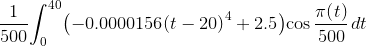We can use Wolfram|alpha for this:

Integral for n = 1 case

Integral for n = 2 case

Hope it helps!

24. Phillip says:

it very much helped - i made the mistake of using 1/x as a function as part of my piece wise function when i could have just used a positive quadratic function to model the same basic curve. Turns out if you go along with 1/x, the math needed to calculate the "an" coefficient is too much for wolfram alpha to compute ...LOL

25. James Jones says:

Hi, could you please explain why we need to use the value 20 in the function expression of the f(t) in f(t) = -0.0000156(t-20)^4 + 2.5??

26. Murray says:

@James: In the "Explanation of the model" section, it says:

The (t − 20) term comes from deciding the curve should start at (0,0), (which makes our lives easier), it will pass through (40,0) since the pulse is 40 ms long, and be centered on t = 20.

That's where the 20 comes from - it's half of the width of the "blip" which goes from 0 to 40.

27. James Jones says:

thank you for the quick reply in the previous comment but can you also explain where you got the period value of 1000ms too? Because I thought the period for the R wave region was 40...

28. Murray says:

The period is 1000 ms because I'm assuming my heart rate is 60 beats per minute, or 1 beat per second.

The R wave "blip" lasts for 40 ms, representing the biggest pumping motion, then has a rest for the next 960 ms (while the heart is doing something else). That pulse repeats every 1000 ms.

29. Dequan says:

Hi Mr. Murray

Could you explain how you did the integration when solving for an and bn because looking at the attached wolframalpha links, I see that the value for the integration when n is 1,2,3,4,... is all -0.039936

Regards, DeQuan

30. Murray says:

@DeQuan: Thanks for alerting me - I realised those Wolfram|Alpha links had a bracket in the wrong place. I've updated them now.

31. Dequan says:

Hmmm I'm sorry but could you please explain how you were able to deduce the integration of an using the integration of each of the n=1,2,3,...?

32. Murray says:

@Dequan: I'm not deducing the integration. I'm just applying the formulas that can be found here: https://www.intmath.com/fourier-series/2-full-range-fourier-series.php

The PDF (linked to in the article) may give you a better idea of what is going on.

33. Dequan says:

Hi,

Is it possible for you to give a clearer explanation in the way that you were able to get the values for the coefficient an? did you generate an arithmetic sequence using the idea that an is simply the sum function for the terms of an? If not, how were u able to get the "n" in the an function?

34. Murray says:

@Dequan: As I mention in the article, even the answer is quite ugly, let alone all the integration steps involved to get to that answer.

Did you have a look at the PDF solution yet? It shows the general terms for the an and bn coefficients. I haven't gone into the algebra involved because this is certainly a place where we should use a computer algebra system and not spend significant chunks of our lives doing it on paper. The integral will involve several integration by parts steps. (see https://www.intmath.com/methods-integration/7-integration-by-parts.php )

The "n" in the Fourier Series expansion just means we need to use integers.

So a0 means we replace all n's with 0,
a1 means we replace all n's with 1,
a2 means replace n's with 2, etc.

You can see how this works in the table in the answer for Example 1 (where I'm finding a0, a1, a2, etc) on this page:

https://www.intmath.com/fourier-series/2-full-range-fourier-series.php

Hope it helps.

35. Ria T says:

Hi,

I wanted to ask if you could possibly share a short tutorial or give an explanation as to how you managed to graph the equation. I am currently using scientific notebook version 6, but whenever I type in the equation obtained and click on graph, the graph simply appears blank. I'm not sure if the equation is too complex for the software to process? I typed in the same equation that you have used but the same keeps happening. Kindly let me know if you know of a possible solution to this issue- if not, it would be great if you could let me know which version of the software you had used in this article.

Thank you,

Ria

36. Murray says:

@Ria: I think I was using a version of Scientific Notebook that had Maple as its processing engine when I wrote that article (and produced the graph outputs). When they changed to MuPad, I was never as happy with the results (both computationally and aesthetically).

One thing I found is that SNB doesn't like square brackets [ ] in expressions (both for computations and graphing). If you have any, try changing them to ordinary parentheses ( ).

Another thing I've tried when facing the "blank graph with no error message to tell you why" problem, is to build up the pieces one at a time until it breaks, then try to rewrite it in some way so it works.

So for example, try to graph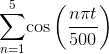Then try to graph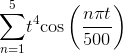Another thing I've resorted to is to expand out inner items (like that integral) first, then substitute that into the expression. So like with humans, if we break it down into its component parts and do those separately, it (might) work!

I tried it again just now in v5.5 using MuPad and it worked fine.

One final thought - it won't handle infinity as the upper limit for the sum! Try n=1 to 5 as a starter.

Good luck.

37. Murray says:

I haven't managed to graph the heart beat successfully yet, but found it was happier with x as the variable, rather than t.

38. Singh says:

Hi, could you please show all the steps involved when you plotted the graph on the scientific notebook because I am unable to plot the graph.
Thanks

39. Murray says:

@Singh: There was nothing special about it - I just clicked the "2D" plot button.

What version of Scientific Notebook are you using? It works fine for me in v5.5, but I also couldn't plot it using v6.

40. Amarnath says:

P wave is caused by the atrial repolarization which is relative to the first half by atrial contraction of right atrium and the second half by contraction of left atrium.
Qrs complex is due to ventricular repolarization
And t wave is due to ventricular repolarization.

41. Thatyougoon says:

Why do you approximate your ECG? You know you can just perform a Fourier transform in discrete time right? Can't you just directly transfer the data collected to a matrix/array and performing Fourier transform on it computationally? I just... I don't know why you went through all this trouble to approximate your data (which resulted in a grave loss of detail), so you could approximate it continuously.

42. Murray says:

@Thatyougoon: The aim (as per the sub-heading in the article) was to model heartbeats in general, producing a periodic function that could be graphed. Doing a Fourier analysis of the real data was not the aim - producing a generalised heartbeat-looking function was.

43. v26 says:

hi MR, I have a question, it is possible to use two signal at the same time? and why?

44. Murray says:

@v26: Fourier series is, in fact, the combination of 2 or more signals at the same time. So it appears the answer to your question would be yes.

45. Julia says:

Hello Sir,

I have been attempting to graph my own heartbeat with Scientific Notebook. However, I am only using the Free Trial (which should be enough). For some reason, I only obtain a straight line when I graph the series for one wave. The function I am working with is f(x)=-(-0.008x+0.324)^2+0.105, the integral boundaries are from 0 to 80. Could you give me instructions as what to do on Scientific Notebook to obtain a satisfactory graph? I would be so thankful.

46. Murray says:

@Julia: Please see my response to Ria above, as she was facing similar problems.

Could you graph your base function successfully? (I mean -(-0.008x+0.324)^2+0.105) As suggested to Ria, start from something that does work, then add complications until it breaks.

Two other thoughts:

(1) I was not impressed with the latest version of Scientific Notebook and did not upgrade my version. (That doesn't help you, I'm sorry, but it may be a version thing - it did work on earlier versions.)

(2) You may also like to try other computer algebra solutions, like SageMath or similar.

47. SM says:

Hi,

Is it possible to model the individual parts of the ECG (P,Q,R,S and T) in order to determine their functions?

48. Murray says:

@SM: Yes indeed, and that's what I'm doing in this article. The PDF has more details about the process.

49. Sarthak says:

Can we use linear piecewise functions in order to model the QRS complex?

50. Murray says:

@Sarthak: I believe you could, but I think the Fourier Series approach would be more appropriate.

51. Sarthak says:

How did you change the x-axis scale in Scientific Notebook (v5.5)?

52. Murray says:

@Sarthak: From the Doing Math with Scientific Notebook PDF:

"From the Axes page of the Plot Properties dialog you can choose the type of axes displayed and the scaling for the axes."

Hope it helps.

53. Jim says:

Interesting article. Have you ever seen a model oscillator for the heart's electrical system?

54. J says:

Hi,

Thank you so much for the above. I just have a quick question. In many other sources the equation for a0 starts with 1/2L and when I tried to derive/prove the a0 equation I also got 1/2L. So I was wondering where the 1/L comes from.

Thank you.

### Comment Preview

HTML: You can use simple tags like <b>, <a href="...">, etc.

To enter math, you can can either:

1. Use simple calculator-like input in the following format (surround your math in backticks, or qq on tablet or phone):
a^2 = sqrt(b^2 + c^2)
(See more on ASCIIMath syntax); or
2. Use simple LaTeX in the following format. Surround your math with $$ and $$.
$$\int g dx = \sqrt{\frac{a}{b}}$$
(This is standard simple LaTeX.)

NOTE: You can mix both types of math entry in your comment.